Get inspired by the success stories of our students in IIT JAM MS, ISI  MStat, CMI MSc DS.  Learn More

# Probability Theory | ISI MStat 2015 PSB Problem B5This is a detailed solution based on Probability Theory of ISI MStat 2015 PSB Problem B5, with the prerequisites mentioned explicitly. Stay tuned for more.

## Problem

Suppose that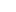and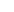are random variables such that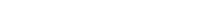Var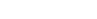Var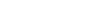(a) Evaluate Cov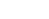.
(b) Show that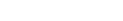.
(c) If in addition, it is given thatis bivariate normal, calculate E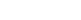.

### Prerequisites

• Basic Probability theory ( Expectation, Variance, and Covariance )
• Normal Distribution
• Gamma Integral

## Solution

(a)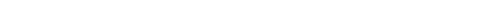Using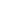, we use Var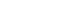- Var=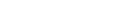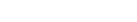.

(b)

Say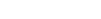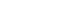.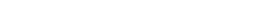.

Do you remember the Cauchy - Schwartz Inequality?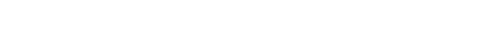. Hence,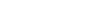.

(c)and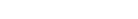.is bivariate normal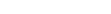~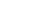.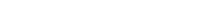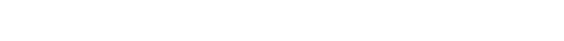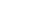.

This is a detailed solution based on Probability Theory of ISI MStat 2015 PSB Problem B5, with the prerequisites mentioned explicitly. Stay tuned for more.

## Problem

Suppose thatandare random variables such thatVarVar(a) Evaluate Cov.
(b) Show that.
(c) If in addition, it is given thatis bivariate normal, calculate E.

### Prerequisites

• Basic Probability theory ( Expectation, Variance, and Covariance )
• Normal Distribution
• Gamma Integral

## Solution

(a)Using, we use Var- Var=.

(b)

Say..

Do you remember the Cauchy - Schwartz Inequality?. Hence,.

(c)and.is bivariate normal~..

This site uses Akismet to reduce spam. Learn how your comment data is processed.

### Knowledge Partner